• Call Now

1800-102-2727•

# Positron- Introduction, Definition, Production, Composition, Mass and Rest Mass, Positron Emission Mammography

Let me tell you a story! During a birthday celebration in my neighbourhood, I met two young boys who looked exactly the same. I got to know that both the children belong to the same parents and they were twins. Do you think that apart from looks, the behaviour of both the children will also be the same? Twins might have similar looks, similar body structures, and similar faces but behaviour might be different and the same was the case with those two young boys. Similarly, there are two types of particles which are emitted in a radioactive process and are known as positron and electron. Though they are produced from the same process still their behaviour and properties are different. In fact, they are counterparts of each other. In 1932, Carl D. Anderson discovered the positron. Let's learn more about positrons and what is the difference between positrons and electrons.

• Introduction to Positron
• Definition of a Positron
• Difference between Positron and Electron
• Production of Positron
• Composition of Positron
• Mass and Rest Mass of the Positron
• Positron Emission Mammography
• Practice Problems
• Frequently Asked Questions - FAQs

## Introduction to Positron

Positron is the type of beta emission which is generally emitted during artificial radioactivity when np value is lower than the required value for nuclear stability. The positron is an antimatter equivalent to that of the electron. When positron decay takes place it results in a decrease in the atomic number by one unit as compared with the parent nuclei. It has a very short life span and loses its kinetic energy when it passes through the matter. It is represented by the symbol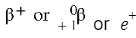. Positron emission and K- electron capture are nearly similar because both the process lowers the number of protons of daughter nuclei by one unit.

When it comes to rest it combines with an electron to form two gamma photons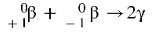.

## Definition of a Positron

A positron can be defined as a subatomic particle that has a mass equal to the mass of an electron but contains a positive charge. It has the same spin as an electron, i.e., ½, but its electrical charge is +1.6 × 10-19C which is +1e. The magnitude of the charge on both electron and positron is equal. Another name for positron is anti-electron.

When an electron and positron collide with each other, destruction occurs that produces two or more photons of gamma rays. This process is termed positron-electron annihilation. Therefore, a positron is simply a positive electron, i.e., it is a subatomic particle that is positively charged and has the same magnitude of charge and mass as that of the electron, thus creating an antiparticle of the negative electron.

Whereas, an electron can be defined as an elementary particle of the atom that has an important role in all the branches of science as well as everyday life. In 1897, English physicist Joseph John Thomson was the first to discover the electron as a subatomic particle. It carries a negative charge and can be free or connected to the atom's nucleus.

## Production of positron

Positron can be produced through two processes:

Pair production: Pair production is the most important example of mass-energy equivalence. A photon makes an electron and a positron in pair formation in such a way that the photon involved in the interaction vanishes throughout the process. We can write the pair production symbolically as:

Photon + photon particle (electron) + anti-particle (positron)

A particle and antiparticle can collide and annihilate each other, generally producing two high-energy gamma rays photons. Pair production obeys the law of conservation of energy and momentum

Particle + antiparticle photon+ photon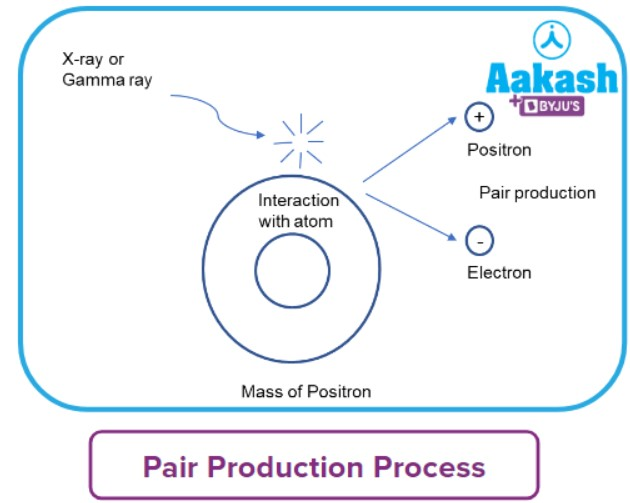Radioactive decay: positrons are formed by departing neutrons in radioactive decay, and their kinetic energy is maintained by their velocity. When the positron collides with other particles, it loses some of its kinetic energy, according to the notion of conservation of energy and momentum. As a result of energy loss, they come to a halt. After losing kinetic energy, the positron particles are ready to participate in an annihilation reaction.

For example;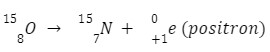## Composition of Positron

A positron has the same mass as that of an electron with an electrical charge of +1e and a spin of 12. It is the antiparticle of the electron. The mass of an electron and positron is the same and is equal to 9.1110-31 kg. Whereas, whereas the mass of the proton which is 1.6610-27 kg. If we consider the ratio of the mass of the proton to the mass of the electron it is approximately 2000 times the mass of an electron and therefore it is considered merely negligible.

## Mass and Rest Mass of Positron

The mass of the electron is 0.000548756 amu. We know that the mass of a positron is the same as that of an electron. Hence, the mass of a position will be 0.000548756 amu or 9.1110-31 kg.

The interaction of a positron with an electron is governed by energy conservation principles. These two particles combine to demolish one another and convert all of their mass to energy, resulting in the formation of two photons because the positron and electron are practically steady before obliteration and remain the same after destruction, the force of the framework is zero on the ground.

Rest mass is the mass of a particle which does not change even when the particle is in motion. For instance, the rest mass of an electron is 9.110-31 kg. Using the famous Einstein equation E= m c2 the rest mass of positron or electron is calculated as 0.511 MeV/c2, where c represent the speed of light.

## Positron Emission Mammography

Positron emission mammography deals with the detection of the cancerous or malignant cell which are highly active in nature and consumes more glucose from the body to perform the metabolic activity. A positron emanation scanner is fundamentally smaller than an expected X- rays beam machine and a PET scanner which helps in determining the biological or metabolic function of tissue or organs of the body.

Isotopes like F-18 are positron producers. Substances composed of these isotopes gather in sickness sites in the body. The cancer patient gets injected with a solution of F-18 fluorodeoxyglucose. The cancer cells absorb this solution, which lights on the position detection screen, providing you with a clear view of the malignant expansion.

## Practice Problems

Q 1. A positron is emitted from. The ratio of mass number and the atomic number of the resulting daughter nuclei is:

a. 13:
b. 11:5
c. 12:6
d. 11:6

We know that positron is represented by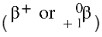and when positron decay takes place it results in a decrease in the atomic number by one unit as compared with the parent nuclei.

Using the above information we get that,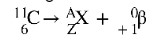‘X’ represents the symbol of the daughter element

‘A’ represents the mass number of the daughter element

‘Z’ represents the atomic number of the daughter element

Equating the mass number and an atomic number of the element on both reactant and product side as it is conserved during a radioactive decay we get,

A=11

6=Z+1

Z=5

Taking the ratio of A and Z, we get;

A:Z=11:5

Q 2. Decrease in the atomic number is observed during the emission of

a. K-capture
b. Positron emission
C. emission
D. Both A and B

Positron is positively charged antiparticle represented by the symbol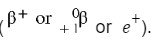. Positron emission and K-electron capture are nearly similarly because both the process lower the number of protons (i.e., atomic number) of daughter nuclei by one unit. Whereas, in the case of

emission, the energy is emitted by the daughter nuclei formed in the excited state during the -decay or -decay and atomic number after the emission is increased by one unit.

Q 3. A radioactive element X has the atomic number 'Z' and mass number ‘A’. Select the correct statement among the following?

a. Both ‘A’ and ‘Z’ of daughter nuclei remain unchanged during positron emission
b. Both ‘A’ and ‘Z’ of daughter nuclei decrease during positron emission
c. Only ‘Z’ of daughter nuclei decreases but ‘A’ remains unchanged during positron emission
d. Only ‘Z’of daughter nuclei increases but ‘A’ remains unchanged during positron emission

Positron is positively charged antiparticle represented by the symbol. When positron decay takes place it results in a decrease in the atomic number by one unit as compared with the parent nuclei without affecting the mass number of the daughter nuclei.

Using the above information, we get that;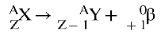Here,

‘X’ represents the symbol of the parent radioactive element

‘Y’ represents the symbol of daughter nuclei

‘A’ represents the mass number of the parent radioactive element and daughter nuclei

‘Z’ represents the atomic number of the parent radioactive element

Q 4. Select the correct option with respect to the positron emission in case of radioactive decay.

a. Both electron and positron have the same mass but a different magnitude of the charge
b. The mass of an electron is more than the mass of a positron but they have the same magnitude of the charge
c. Both mass and magnitude of the charge are same for positron and electron.
d. Mass of a positron is more than the mass of a proton but they have the same magnitude of charge

A positron can be defined as a subatomic particle that has a mass equal to the mass of an electron (i.e., 9.1110-31 kg). Positron is a positively charged particle and has the same spin as an electron, i.e., ½, but its electrical charge is +1e . Electron is a negatively charged particle though their magnitude is the same.

So, both mass and magnitude of the charge are the same for positron and electron.

Q 1.How we can differentiate positron and electron?
The difference between Positron and Electron are:

• Positrons and electrons both are β (beta) particles, but the electron is β- while the positron is its counterpart β+.
• When a positron undergoes emission, a nucleus is formed, which has the same mass number, but the atomic number is one unit less than the previous one, whereas in an electron emission, an atomic number is one greater than the previous one.
• β-emission can be defined as the process of the emission of a β particle by the nucleus, which can either be an electron or positron. This emission of the nucleus helps the atom to reach close to the optimum value of np of nucleus placed along the nuclear stability belt.

Q 2. What is the difference between positron and neutrino?
Positron decay is a form of beta decay that occurs in radioactive atoms. It is produced when a proton in the nucleus is transformed into a neutron which has a mass equal to the mass of an electron but the charge opposite to that of an electron. Whereas, neutrinos, which are similar to electrons have very less mass approximately close to zero and do not have a charge. Neutrino is formed when the atomic part comes closer to each other and dissociates into stable nuclei.

Q 3. Which isotopes can undergo positron emission?
Answer: Positron is the type of beta emission which is generally emitted during artificial radioactivity when np the value is lower than the required value for nuclear stability. Few isotopes which can undergo positron emission are: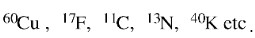Q 4. Is there any difference between positron and proton?
Answer: Positron and proton both are positively charged particles with an electrical charge +1e but, the mass of the proton is  1.6610-27 kg which is approximately 2000 times heavier than the mass of positron which has a mass of 1.6610-27 kg.

Related topics

 Nuclear reaction Rate of radioactive decay Radiocarbon dating Nuclear stability Group displacement law and its limitation Beta and gamma rays Radioactivity Nuclear reactor Types of nuclear reactionsTalk to our expert
Resend OTP Timer =
By submitting up, I agree to receive all the Whatsapp communication on my registered number and Aakash terms and conditions and privacy policy